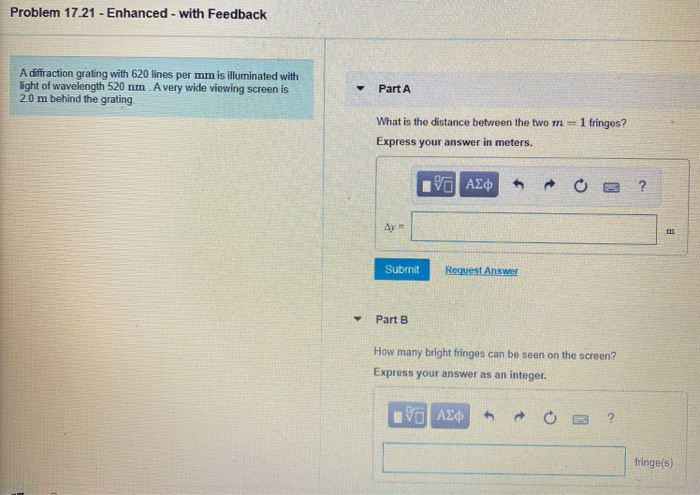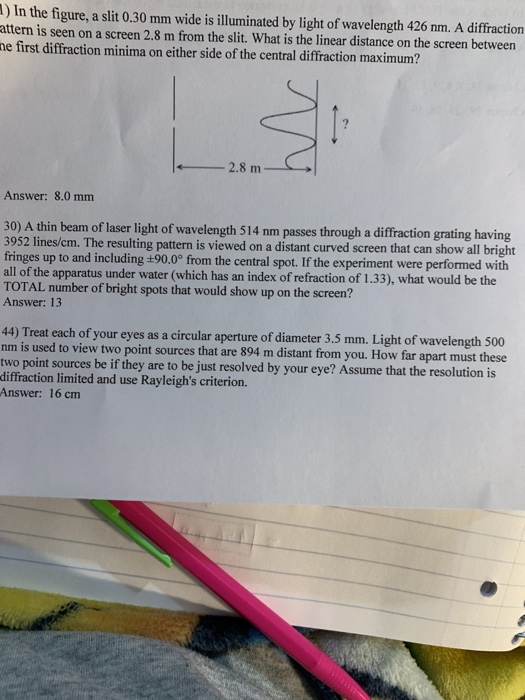Question

# A diffraction grating with 600 lines/mm is illuminated with light of wavelength 510 nm. A very...

A diffraction grating with 600 lines/mm is illuminated with light of wavelength 510 nm. A very wide viewing screen is 4.2 m behind the grating.

Part A

What is the distance between the two m = 1 bright fringes?

Δy =

Part B

How many bright fringes can be seen on the screen?

N =

(A) d = 1 x 10^-3 m / 600 = 1.67 x 10^-6

y = m lambda D / d

y = (1 x 510 x 10^-9 x 4.2) / (1.67 x 10^-6)

y = 1.2852 m

d = 2 y = 2.57 m

(B) sin(theta) = m lambda / d

sin90 = m (510 x 10^-9) / (1.67 x 10^-6)

m = 3.26

total bright fringe = 2(3) + 1 = 7

#### Earn Coins

Coins can be redeemed for fabulous gifts.

Similar Homework Help Questions
• ### A 480 lines/mm diffraction grating is illuminated by light of wavelength 510 nm . How many...

A 480 lines/mm diffraction grating is illuminated by light of wavelength 510 nm . How many bright fringes are seen on a 4.0-m-wide screen located 2.1 m behind the grating?

• ### A diffraction grating with 610 lines per mm is illuminated with light of wavelength 520 nm...

A diffraction grating with 610 lines per mm is illuminated with light of wavelength 520 nm . A very wide viewing screen is 2.0 m behind the grating. Part A What is the distance between the two m=1 fringes? Express your answer in meters. ΔyΔ y = nothing m Request Answer Part B How many bright fringes can be seen on the screen? Express your answer as an integer.

• ### Problem 17.21 - Enhanced - with Feedback A diffraction grating with 620 lines per mm is...Problem 17.21 - Enhanced - with Feedback A diffraction grating with 620 lines per mm is illuminated with light of wavelength 520 nm A very wide viewing screen is 2.0 m behind the grating Part A What is the distance between the two m=1 fringes? Express your answer in meters. ΡΙ ΑΣφ ? Ay = n Submit Request Answer Part B How many bright fringes can be seen on the screen? Express your answer as an integer. Η ΑΣφ fringe(s)

• ### A 500 lines per mm diffraction grating is illuminated by light of wavelength 510...continues

A 500 lines per mm diffraction grating is illuminated by light of wavelength 510 nm. What is the angle of each diffraction order?

• ### A laser beam of wavelength 750 nm shines through a diffraction grating that has 750 lines/mm...

A laser beam of wavelength 750 nm shines through a diffraction grating that has 750 lines/mm and observed on a screen 1.4 m behind the grating. Part A How many bright fringes can be observed on a screen?

• ### A 500 lines per mm diffraction grating is illuminated by light of wavelength 560 nm ....

A 500 lines per mm diffraction grating is illuminated by light of wavelength 560 nm . Part A What is the maximum diffraction order seen Part B: What is the angle of each diffraction order starting from zero diffraction order to the maximum visible diffraction order? Enter your answers in degrees in ascending order separated by commas.

• ### A 500 lines per mm diffraction grating is illuminated by light of wavelength 640 nm .

A 500 lines per mm diffraction grating is illuminated by light of wavelength 640 nm . For the steps and strategies involved in solving a similar problem, you may view a Video Tutor Solution.Part AWhat is the maximum diffraction order seen? Express your answer as an integer. Part B What is the angle of each diffraction order starting from zero diffraction order to the maximum visible diffraction order?

• ### ) In the figure, a slit 0.30 mm wide is illuminated by light of wavelength 426 nm. A diffraction attern is seen on a screen 2.8 m from the slit. What is the linear distance on the screen betw...) In the figure, a slit 0.30 mm wide is illuminated by light of wavelength 426 nm. A diffraction attern is seen on a screen 2.8 m from the slit. What is the linear distance on the screen between e first diffraction minima on either side of the central diffraction maximum? Answer: 8.0 mm 30) A thin beam of laser light of wavelength 514 nm passes through a diffraction grating having 3952 lines/cm. The resulting pattern is viewed on a...

• ### A diffraction grating with an unknown number of lines per mm, is illuminated by light at...

A diffraction grating with an unknown number of lines per mm, is illuminated by light at a wavelength l = 633 mm, and the first order diffraction pattern is seen at an angle β= 45.OO. Light of a second wavelength is shone on to the grating, whose second order diffraction pattern is seen at l = 62.00. What is the wavelength of the second beam?

• ### Light of wavelength 429 nm (in vacuum) is incident on a diffraction grating that has a...

Light of wavelength 429 nm (in vacuum) is incident on a diffraction grating that has a slit separation of 1.2 × 10-5 m. The distance between the grating and the viewing screen is 0.10 m. A diffraction pattern is produced on the screen that consists of a central bright fringe and higher-order bright fringes (see the drawing). (a) Determine the distance y from the central bright fringe to the second-order bright fringe. (Hint: The diffraction angles are small enough that...